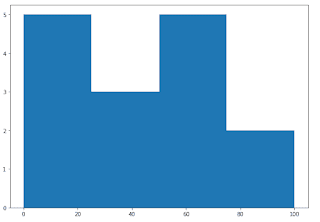# HSSlive: Plus One & Plus Two Notes & Solutions for Kerala State Board

## How to Draw a Histogram Using Python Turtle, Tkinter, Matplotlib & Without Libraries

How to Draw a Histogram in Python - There are various methods to create a Histogram using python libraries like turtle, tkinter and matplotlib. Also, you will learn how to make a Histogram using python in easy and simple steps. We have also provided the python code for drawing a Histogram pattern. If you have introductory to intermediate knowledge in Python and statistics, then you can use this article as a one-stop shop for building and plotting Histogram patterns in Python using libraries from its scientific stack, including NumPy, Matplotlib, Pandas, and Seaborn.

Plotting a Histogram in python is a simple procedure, and python in its simplest provides multiple easy methods to do so.

## How to Draw a Histogram using Python?

Given below is the process to draw a Histogram in python using libraries like Turtle, Tkinter, Matplotib and others.

Four Method to make a Histogram in Python using multiple libraries:

1. How to draw a Histogram using python turtle?
2. How to draw a Histogram using python tkinter?
3. How to draw a Histogram using python matplotlib?
4. How to draw a Histogram using python code without library?

## How to Draw a Histogram using Python Turtle?

Check below step by step process to make a Histogram using the python turtle library.

Step

Code

import turtle

def drawBar(t, height):
""" Get turtle t to draw one bar, of height. """
t.begin_fill() # start filling this shape
t.left(90)
t.forward(height)
t.write(str(height))
t.right(90)
t.forward(40)
t.right(90)
t.forward(height)
t.left(90)
t.end_fill() # stop filling this shape

xs = [48, 117, 200, 240, 160, 260, 220] # here is the data
maxheight = max(xs)
numbars = len(xs)
border = 10

wn = turtle.Screen() # Set up the window and its attributes
wn.setworldcoordinates(0-border, 0-border, 40*numbars+border, maxheight+border)
wn.bgcolor("lightgreen")

tess = turtle.Turtle() # create tess and set some attributes
tess.color("blue")
tess.fillcolor("red")
tess.pensize(3)

for a in xs:
drawBar(tess, a)

wn.exitonclick()

OutputHow to Draw a Histogram Using Python Turtle, Tkinter, Matplotlib & Without Libraries

## How to Draw a Histogram using Python Tkinter?

Check below step by step process to make a Histogram using the python tkinter library.

Step

Code

Output

## How to Draw a Histogram using Python Matplotlib?

Check below step by step process to make a Histogram using the python matplotlib library.

Step

Step 1: Install the Matplotlib package'

Step 2: Collect the data for the histogram

Step 3: Determine the number of bins

Step 4: Plot the histogram in Python using matplotlib

Code

from matplotlib import pyplot as plt
import numpy as np

# Creating dataset
a = np.array([22, 87, 5, 43, 56,
73, 55, 54, 11,
20, 51, 5, 79, 31,
27])

# Creating histogram
fig, ax = plt.subplots(figsize =(10, 7))
ax.hist(a, bins = [0, 25, 50, 75, 100])

# Show plot
plt.show()

Output

## How to Draw a Histogram using Python Code without Library?

Step

Code

Output

Note: Also, these codes might not run in an online compiler please use an offline compiler. Programmers can make any changes in the code according to their specific requirement.

## Conclusion on How to Draw a Histogram using Python

We hope the programs and methods provided above on how to make a Histogram using python libraries have been helpful to you. If there is any issue in any of the code please let us know in the comments. We will try to resolve it as soon as possible.

Share: